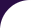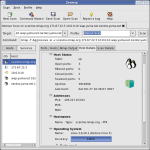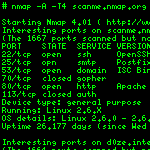Intro Reference Guide Book Install Guide Download Changelog Zenmap GUI Docs Bug Reports OS Detection Propaganda Related Projects In the Movies In the News## OS Matching Algorithms

### IPv4 matching

Nmap's algorithm for detecting matches is relatively simple. It takes a subject fingerprint and tests it against every single reference fingerprint in `nmap-os-db`.

When testing against a reference fingerprint, Nmap looks at each probe category line from the subject fingerprint (such as `SEQ` or `T1`) in turn. Any probe lines which do not exist in the reference fingerprint are skipped. When the reference fingerprint does have a matching line, they are compared.

For a probe line comparison, Nmap examines every individual test (`R`, `DF`, `W`, etc.) from the subject category line in turn. Any tests which do not exist in the reference line are skipped. Whenever a matching test is found, Nmap increments the `PossiblePoints` accumulator by the number of points assigned to this test. Then the test values are compared. If the reference test has an empty value, the subject test only matches if its value is empty too. If the reference test is just a plain string or number (no operators), the subject test must match it exactly. If the reference string contains operators (`|`, `-`, `>`, or `<`), the subject must match as described in the section called “Test expressions”. If a test matches, the `NumMatchPoints` accumulator is incremented by the test's point value.

Once all of the probe lines are tested for a fingerprint, Nmap divides `NumMatchPoints` by `PossiblePoints`. The result is a confidence factor describing the probability that the subject fingerprint matches that particular reference fingerprint. For example, `1.00` is a perfect match while `0.95` is very close (95%).

Test point values are assigned by a special `MatchPoints` entry (which may only appear once) in `nmap-os-db`. This entry looks much like a normal fingerprint, but instead of providing results for each test, it provides point values (non-negative integers) for each test. Tests listed in the `MatchPoints` structure only apply when found in the same test they are listed in. So a value given for the `W` (Window size) test in `T1` doesn't affect the `W` test in `T3`. A test can be effectively disabled by assigning it a point value of 0. An example `MatchPoints` structure is given in Example 8.10.

Example 8.10. The `MatchPoints` structure

```MatchPoints
SEQ(SP=25%GCD=75%ISR=25%TI=100%CI=50%II=100%SS=80%TS=100)
OPS(O1=20%O2=20%O3=20%O4=20%O5=20%O6=20)
WIN(W1=15%W2=15%W3=15%W4=15%W5=15%W6=15)
ECN(R=100%DF=20%T=15%TG=15%W=15%O=15%CC=100%Q=20)
T1(R=100%DF=20%T=15%TG=15%S=20%A=20%F=30%RD=20%Q=20)
T2(R=80%DF=20%T=15%TG=15%W=25%S=20%A=20%F=30%O=10%RD=20%Q=20)
T3(R=80%DF=20%T=15%TG=15%W=25%S=20%A=20%F=30%O=10%RD=20%Q=20)
T4(R=100%DF=20%T=15%TG=15%W=25%S=20%A=20%F=30%O=10%RD=20%Q=20)
T5(R=100%DF=20%T=15%TG=15%W=25%S=20%A=20%F=30%O=10%RD=20%Q=20)
T6(R=100%DF=20%T=15%TG=15%W=25%S=20%A=20%F=30%O=10%RD=20%Q=20)
T7(R=80%DF=20%T=15%TG=15%W=25%S=20%A=20%F=30%O=10%RD=20%Q=20)
U1(R=50%DF=20%T=15%TG=15%TOS=0%IPL=100%UN=100%RIPL=100%RID=100%RIPCK=100%RUCK=100%RUL=100%RUD=100)
IE(R=50%DFI=40%T=15%TG=15%TOSI=0%CD=100%SI=100%DLI=100)
```

Once all of the reference fingerprints have been evaluated, Nmap orders them and prints the perfect matches (if there aren't too many). If there are no perfect matches, but some are very close, Nmap may print those. Guesses are more likely to be printed if the `--osscan-guess` option is given.

### IPv6 matching

IPv6 OS classification uses a machine learning technique called logistic regression. Nmap uses the LIBLINEAR library to do this classification. The process starts with a large corpus of training examples, which are fingerprints submitted by Nmap users and carefully labeled with their OS. Each training example is represented by a feature vector, which can be thought of as the coordinates of that OS in a multi-dimensional space. The training algorithm calculates an optimal boundary between members of each OS class and members of every other class. It then encodes each of these boundaries as a vector. There is a different vector for each OS class.

When matching, the engine takes each of these boundary vectors in turn and calculates a dot product between it and the feature vector. The result is a single real number. The higher (more positive) the number, the more likely the match. Negative numbers are unlikely matches. A number x is mapped from the range [−∞, ∞] to [0, 100] using the logistic formula 100 / (1 + ex). (This is the source of the name logistic regression.)

In general, the OS class with the highest score is the most likely match, but in the case of a never-before-seen operating system, it's possible to have a very high score but an inaccurate match nevertheless. Therefore a second novelty detection algorithm checks whether the observed fingerprint is very unlike the other representatives of the class. The algorithm finds the Euclidean distance from the observed feature vector to the mean of the feature vectors of the members of the class, scaled in each dimension by the inverse of that feature's variance. Feature vectors similar to those already seen will have low novelty, and those that are different will have high novelty.

The OS class with the highest score is reported as a match, but only if the novelty is below 15. Also, if the two highest OS classes have scores that differ by less than 10%, the classification is considered ambiguous and not a successful match. Sample logistic and novelty scores from a run against Mac OS X 10.6.8 are shown in Table 8.9, “OS guesses against Mac OS X”.

Table 8.9. OS guesses against Mac OS X

ScoreNoveltyOS class
61.05%1.00Apple Mac OS X 10.6.8 - 10.7.0 (Snow Leopard - Lion) (Darwin 10.8.0 - 11.0.0)
10.08%18.04Apple Mac OS X 10.7 (Lion) (Darwin 11.1.0)
9.97%24.06Apple Mac OS X 10.6.8 (Snow Leopard) (Darwin 10.8.0)
e)
9.43%19.26Apple Mac OS X 10.7.2 (Lion) (Darwin 11.2.0)
5.99%23.63Apple Mac OS X 10.4.11 (Tiger) (Darwin 8.11.1)
2.28%34.67Apple iPhone mobile phone (iOS 4.2.1)
2.19%35.07Apple Mac OS X 10.4.7 (Panther) (Apple TV 3.0.2)
2.19%57.63HP ProCurve 2520G switch
2.04%37.03Apple Mac OS X 10.6.8 (Snow Leopard) (Darwin 10.8.0)
2.03%68.55Apple Mac OS X 10.6.8 (Snow Leopard) (Darwin 10.8.0)
0.59%79.61FreeBSD 6.1-RELEASE

There isn't a separate data file containing the IPv6 OS database as there is with IPv4. The database is stored in C++ source code file `FPModel.cc`. This file contains scaling constants (used to put feature values roughly into the range [0, 1]), and the boundary vectors described above.

[ Nmap | Sec Tools | Mailing Lists | Site News | About/Contact | Advertising | Privacy ]